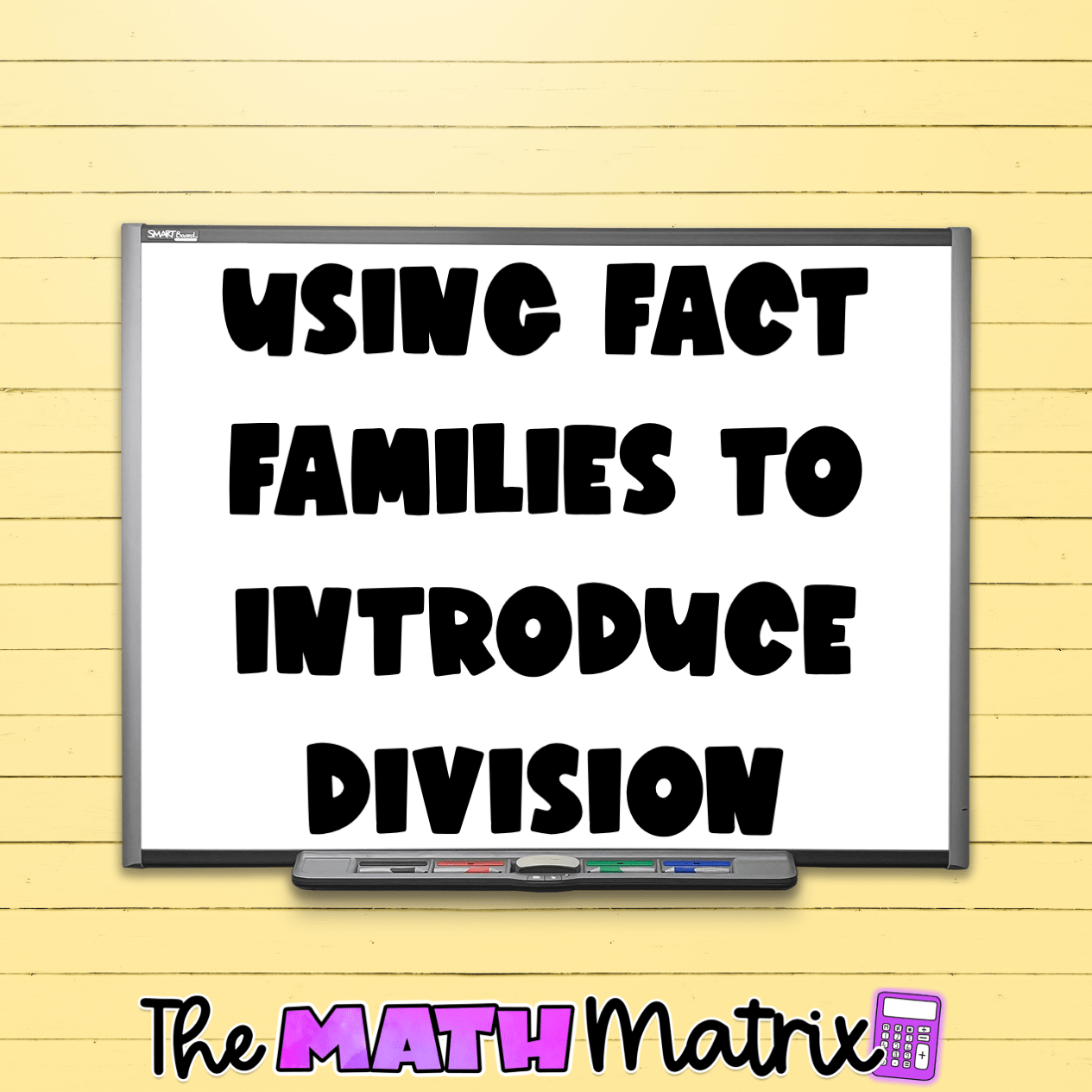# Using Fact Families to Introduce DivisionUtilizing fact families creates a strong mathematical foundation. Your students might have explored fact families for addition and subtraction, but they are just as important to understand the connection between multiplication and division for your upper elementary students. Prior to starting my division unit, I consistently reinforce multiplication and division fact families.

###### What Are fact Families?

Fact families are a set of three numbers that form a group of multiplication and division facts. For example, 4, 5, and 20 form a fact family, since we can write four different equations:

1. 4 x 5 = 20
2. 5 x 4 = 20
3. 20 ÷ 4 = 5
4. 20 ÷ 5 = 4

With three numbers, there are exactly four equations – two multiplication equations and two division equations.

The same rang true for addition and subtraction fact families! When I ask my students what a fact family is before introducing it with our new chapter, they always mention adding and subtracting first! They are so mesmerized when I introduce the same concept with the two newer operations in their repertoire – multiplication and division.

###### Why are Fact Families Important?

Introducing fact families prior to starting division is one of my favorite ways to reinforce the commutative property of multiplication. Even if the factors are in a different order, the product will be the same. As a visual, I like to show the different arrays a fact family makes. In the example of 4, 5, and 20: 4 groups of 5 produces a different array than 5 groups of 4, yet they both have 20 objects in all.

It’s the same thing if you have 20 objects and split them into 4 groups with 5 objects in each group OR into 5 groups with 4 objects in each group.

This visual helps illustrate the relationship between multiplying and dividing numbers. After reviewing fact families with my students, I always like to practice them. Yes, some students find the practice redundant, but it is important regardless to understand WHY it works. By understanding the basic fact families and WHY they work will help students understand WHY we can check our problems and explore inverse operations.